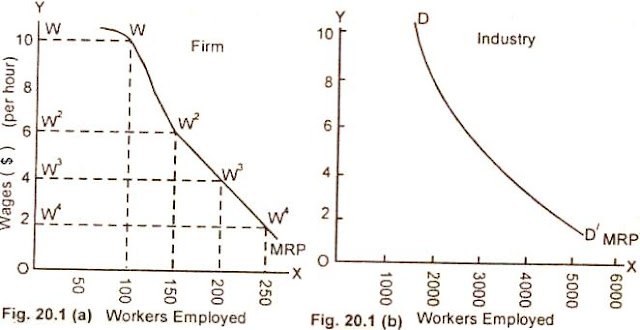### MODERN THEORY”OF WAGES

MODERN THEORY”OF WAGES
Wage determination under perfect competition .
The modern economists are of the view that just as the price of a commodity is determined by the interaction of the forces of demand and supply, the wage rate of specific types of workers can also be determined in the same way with the help of usual demand and supply analysis. Let us discuss in brief as to what do we
mean by demand for and supply of labour as used in determination of wage rates.
Demand for labour
Demand for labour is a derived demand. It is derived from the demand of goods which it produces ,along with other factors of production. Under conditions of perfect competition, the workers engaged in specific types of occupations receive a wage rate which is equal to the value of the marginal revenue product of specific type of labour. The marginal revenue product of a labour (MRP) means the addition to the ,firm’s revenue which it obtains by hiring one additional worker.
Marginal Revenue Productivity (MRP). An employer hires labour in order to make profit. He, while employing a worker, compares the cost of hiring a worker to the contribution he is expected to make to the total revenue of the firm. So long as the addition made by the labour to the revenue is greater than .the cost of employing him, the entrepreneur will engage that labour. In other words, we can say that so long as the marginal revenue product of labour is higher than the cost of employing him, the employer employs that worker. The entrepreneur will continue hiring the worker upto the point at which the cost of employing a worker is just equal to the marginal revenue product of the labour.
The marginal revenue productivity Of labour, due to the operation of law of diminishing returns decreases as more workers are put to work. Thus the demand curve for labour is downward, sloping (The demand curve for labour is the MRP curve of the firm as each worker of same skill and efficiency earns what his labour is worth). If we add up the demand curves for labour of all the individual firms (the MRP curves) we get the demand curve of the industry. It is the demand of the industry which determines wage rate for labour. The individual firm in a competitive market has to accept wage rate set in the market.The downward sloping marginal revenue product (MRP) curve is the demand curve, for labour and is shown in figure 20.1(a). The industry demand curve for labour which is the sum of the individual demand curves of particular tylpes of individual firms is shown inIn a competitive labour market as shown in diagram 20.1(a) a firm employs 100 worker of same skill at a wage rate of Rs. .10 each per hour and 250 workers at the wage rate of Rs. two per hour. The MRP curve of the firm is down sloping.
The industry demand curve for labour which is the sum of the demand curves of individual firm is also down sloping as shown in figure 21.1(b). Here the total demand of all the identical firms in the market is 2000 at the wage rate of Rs. 10 per hour and 5000 workers at the wage rate of Rs. two per hour.